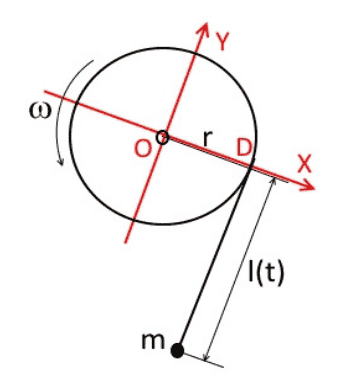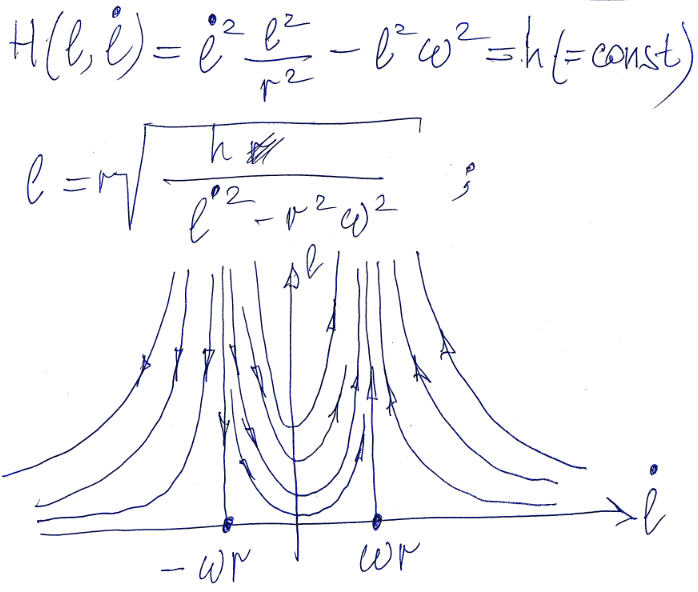In b). Since the force T is perpendicular to the trajectory of the mass m, T does not perform any work on m, therefore the translational mechanical energy is conserved, from which I deduce that the initial speed is equal to the final speed, moreover, the speed is constant.

Now, when analyzing the linear momentum, due to the force on m T, it can be deduced that when evaluating the integral of this force in time, it gives different from 0, but since the speed of the mass m remains constant as well as its mass, its linear momentum must remain constant, so the previous integral would be equal to 0 ... can you see this possible contradiction?

I am right?

\begin{equation*}
\int T(t)\,\mathrm{d}t=0?
\end{equation*}

#### Attachments

•d.png
39.5 KB · Views: 83

Mentor
since the speed of the mass m remains constant as well as its mass, its linear momentum must remain constant,
This is incorrect. Momentum is a vector quantity. It can change without its magnitude changing.

•Thank you so much! i have overlooked it

This is incorrect. Momentum is a vector quantity. It can change without its magnitude changing.
Thank you so much! i have overlooked it

•Dale
Livio Arshavin Leiva
If the radial distance of the ball to the post is reduced, and it does since eventually it hits the post, that means a translation exists in radial direction.

In case (a), the tension of the string T is also in the radial direction, so this force is performing some work on the ball, because the ball has some translation also in the radial direction. But, since this force passes through the center of the post, it can not perform any torque on the ball, and thus the angular momentum with respect to the center of the post is a conserved quantity. If you calculate angular momentum conservation, you will reach that the magnitude of the speed v is higher for a smaller radius, thus the kinetic energy (proportional to v^2) has increased. The energy for this increase is provided by the work performed by the force T. (T is not constant, though, for smaller radius it also increases) Please note that, even though T is in the radial direction, the speed of the ball is not always perpendicular to this force, because in order to reduce the radius, it needs some component of the speed in the radial direction. You can imagine the trajectory of the ball as some kind of spiral. Then, the radial force T is not exactly perpendicular to the trajectory, and this is why the magnitude of the speed of the ball increases. However, the final speed of the ball, since angular momentum is conserved, does not depend on the specific trajectory the ball follows.

In case (b), the tension T is also not exactly perpendicular to the speed of the ball, but it's more clear now since the size of the post R is finite. According to the drawing, there's a component of T that is opposite to the speed direction. Also, now T does not pass through the center of the post, so the angular momentum is not conserved any more, this is, a torque is produced by T in the system. The calculation of the final speed looks harder in this case.

•In case (b), the tension T is also not exactly perpendicular to the speed of the ball
It is exactly perpendicular to the velocity of the ball and the kinetic energy is conserved

•Livio Arshavin Leiva
It is exactly perpendicular to the velocity of the ball and the kinetic energy is conserved
Then, angular momentum is not conserved? How can the radius change if the speed is never in radial direction? If the energy is conserved, what happens with the work done by T?

•Then, angular momentum is not conserved?
if you mean the angular momentum about the axis of the cylinder then yes, it is not conserved
If the energy is conserved, what happens with the work done by T?
this work is equal to zero

•Livio Arshavin Leiva
if you mean the angular momentum about the axis of the cylinder then yes, it is not conserved
Sorry, I thougth it was case (a). My bad. How can you prove that in case (b) the kinetic energy is conserved?

How can you prove that in case (b) the kinetic energy is conserved?
I calculated the velocity and made sure that it is perpendicular to the rope

Livio Arshavin Leiva
I calculated the velocity and made sure that it is perpendicular to the rope
Can I see the calculation?

Homework Helper
Then, angular momentum is not conserved? How can the radius change if the speed is never in radial direction? If the energy is conserved, what happens with the work done by T?
We are in case b here with the cord wrapping around the pipe. I will assume that by "radial direction" you mean the direction toward the center of the pipe. Importantly, this is not the same as the direction along the cord.

The object is moving at right angles to the cord. It is not moving at right angles to the radial direction. It is making progress in the radial direction.

You ask about the work done by T. What work done by T? The object is not making progress parallel to the cord. It is making progress parallel to the radial direction. They are not the same thing.

•Livio Arshavin Leiva
We are in case b here with the cord wrapping around the pipe. I will assume that by "radial direction" you mean the direction toward the center of the pipe. Importantly, this is not the same as the direction along the cord.

The object is moving at right angles to the cord. It is not moving at right angles to the radial direction. It is making progress in the radial direction.

You ask about the work done by T. What work done by T? The object is not making progress parallel to the cord. It is making progress parallel to the radial direction. They are not the same thing.
I thought it was case (a), sorry.

The problem becomes interesting if in the case (b) the pipe rotates about its axis with given angular velocity ##\omega=const## and the question is will the ball reach the pipe or not?

•Livio Arshavin Leiva and Dale
Livio Arshavin Leiva
It is exactly perpendicular to the velocity of the ball and the kinetic energy is conserved
I was confused on what the statement of the problem meant by "tangentially". At first I thought it meant perpendicular to a line from the center of the post to the ball, but I guess it really meant simply perpendicular to the string. Because in case (b) the velocity of the ball is constrained by the string so it can not have a component parallel to the string pointing outside, so the initial velocity couldn't have been as what at first I understood as "tangentially"...
So in the two cases the initial velocity have a slightly different direction, which is not so good for comparative purposes...

Homework Helper
So in the two cases the initial velocity have a slightly different direction, which is not so good for comparative purposes...
Yes, a slight ugliness to the problem. But negligible as long as r is large compared to R.

•Livio Arshavin Leiva
Livio Arshavin Leiva
The problem becomes interesting if in the case (b) the pipe rotates about its axis with given angular velocity ##\omega=const## and the question is will the ball reach the pipe or not?
If ##\omega=v_0/r##, then the ball should rotate at a fixed distance ##r## with the string not wrapping nor unwrapping around the post, right?

Homework Helper
If ##\omega=v_0/r##, then the ball should rotate at a fixed distance ##r## with the string not wrapping nor unwrapping around the post, right?
If the post is rotating then the cord will be moving in the direction of its length. This results in a transfer of energy to or from the ball. One could write down a formula for the rate of transfer. The ball cannot maintain a fixed rotation rate if it is speeding up or slowing down at a fixed distance from the post.

Last edited:
If ##\omega=v_0/r##, then the ball should rotate at a fixed distance ##r## with the string not wrapping nor unwrapping around the post, right?
The Lagrangian is
$$L(l,\dot l)=\frac{m}{2}\Big(l^2\omega^2+\frac{l^2}{r^2}\Big(\frac{dl}{dt}\Big)^2\Big)$$If ##\omega=v_0/r##, then the ball should rotate at a fixed distance ##r## with the string not wrapping nor unwrapping around the post, right?
Yes sir, that is true, but in this problem that does not happen. Even so, due to the conservation of kinetic energy, it is not necessary to analyze the behavior of mass m during its anneal to find its final speed. I think the essence of this problem is to analyze what quantities are conserved and to use this powerful tool to avoid complex developments.

f the post is rotating then the cord will be moving in the direction of its length. This results in a transfer of energy to or from the ball. One could write down a formula for the rate of transfer. The ball cannot maintain a fixed rotation rate if it is speeding up or slowing down at a fixed distance from the post.
Try to draw a phase portrait of the system in the plane ##(l,\dot l)##.(See #19) You will be surprised I promise

Livio Arshavin Leiva
The Lagrangian is
$$L(l,\dot l)=\frac{m}{2}\Big(l^2\omega^2+\frac{l^2}{r^2}\Big(\frac{dl}{dt}\Big)^2\Big)$$

View attachment 266954
What's the procedure to get to that lagrangian?

What's the procedure to get to that lagrangian?
To calculate the kinetic energy of the ball as a function of ##l,\dot l##.

Livio Arshavin Leiva
To calculate the kinetic energy of the ball as a function of ##l,\dot l##.
I know but I couldn't reach that expression...

I know but I couldn't reach that expression...

#### Attachments

• disk_eng.pdf
138.6 KB · Views: 77
•Livio Arshavin Leiva and etotheipi
Livio Arshavin Leiva
Very clear. Thank you.